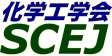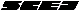## \$B9V1i%W%m%0%i%`!J2q>l!&F|DxJL!K(B

### S\$B2q>l(B \$BBh(B2\$BF|(B

\$B:G=*99?7F|;~!'(B2012-08-20 15:02:17
\$B9V1i(B
\$B;~9o(B
\$B9V1i(B
\$BHV9f(B
\$B9V1iBjL\!?H/I=\$B%-!<%o!<%I(B\$BJ,N`(B
\$BHV9f(B
\$B\$BHV9f(B
\$B%7%s%]%8%&%`(B \$B!c5\$K"!&1UE)!&HyN3;RJ,;69)3X(B2012\$B!d(B
(9:40\$B!A(B10:40)\$B!!(B(\$B:BD9(B \$B@6Ln(B \$BJ8M:(B)
9:40\$B!A(B 10:00S203Korteweg\$BNO\$K\$h\$C\$F<+H/E*\$K1?F0\$9\$k1UE)\$NJQ7A%@%\$%J%_%/%9(B
(\$B:eBg4p9)(B) \$B!{(B(\$B@5(B)\$BH<(B \$B5.I'(B \$B!&(B \$B@P0f(B \$BN< \$B!&(B \$BKRLn(B \$B>4B@(B \$B!&(B (\$B@5(B)\$B9bLZ(B \$BMNJ?(B \$B!&(B (\$B@5(B)\$B2,Ln(B \$BBYB'(B
Korteweg force
Aqueous two-phase system
Self-propelled motion of droplet
S-2867
10:00\$B!A(B 10:20S204\$B?eJ?@E;_N.BNAX\$HC10l1UE)\$N9g0l;~4V(B
(\$B;37ABg1!M}9)(B) \$B!{(B(\$B3X(B)\$BF`NI(B \$B@i9,(B \$B!&(B (\$B@5(B)\$BLg3p(B \$B=( \$B!&(B (\$B@5(B)\$B86ED(B \$B1QFs(B \$B!&(B (\$B@5(B)\$B7*;3(B \$B2mJ8(B
Drop coalescence
Coalescence time
Liquid-liquid system
S-2825
10:20\$B!A(B 10:40S205\$BQrCGN.Cf\$r>e>:\$9\$k1UE)\$K:nMQ\$9\$kMHNO\$K4X\$9\$k8&5f(B
(\$BFAEgBg1!(BSTS\$B8&(B) \$B!{(B(\$B@5(B)\$BB@ED(B \$B8w9@(B \$B!&(B (\$B< \$B!&(B (\$B@5(B)\$B5HED(B \$BK-(B
Shear flow
Drop rise motion
Lift force
S-28644
(10:40\$B!A(B11:40)\$B!!(B(\$B:BD9(B \$B;{:d(B \$B9(0l(B)
10:40\$B!A(B 11:00S206\$BLO5<5\$K"\$rMQ\$\$\$?:.Aj7OM"AwFC@-\$K4X\$9\$k8&5f(B
(\$B \$B!&(B \$BEb?N86(B \$B9';K(B \$B!&(B (\$B@5(B)\$BE{0f(B \$B=SM:(B
laser induced fluorescence
temperature measurement
multiphase
S-28838
11:00\$B!A(B 11:20S207\$B3&LL3h@-:^IU2C\$K\$h\$k5\$K"1?F0JQ2=\$HJ*
(\$B@EBg1!(B) \$B!{(B(\$B3X(B)\$BD;@8(B \$B2mI'(B \$B!&(B (\$B@EBgAO2J1!(B) (\$B@5(B)\$Bc7F#(B \$BN4G7(B
mass transfer
surfactant
CO2 bubble
S-28286
11:20\$B!A(B 11:40S208MH\$BHoJ\$5\$K"\$H%a%?%s5\$K"\$N5sF0\$NAj0cE@\$K\$D\$\$\$F(B
(\$B;:Am8&(B) (\$B6&(B)\$B:4F#(B \$B9/@2(B \$B!&(B \$B!{(B(\$B@5(B)\$B@6Ln(B \$BJ8M:(B \$B!&(B (\$B6&(B)\$B>.3^86(B \$B7<0l(B \$B!&(B (\$B@5(B)\$B;3K\(B \$B2B9'(B \$B!&(B (\$BEl3\$Bg(B) (\$B6&(B)\$B@6?e(B \$B2lG7(B
Methane Hydrate
Bubble
Zig zag
S-28456

\$B9V1i%W%m%0%i%`(B
\$B2=3X9)3X2q(B \$BBh(B44\$B2s=)5(Bg2q(B(C) 2012 \$B8x1W
Most recent update: 2012-08-20 15:02:17
For more information contact \$B2=3X9)3X2qElKL;YIt(B \$BBh(B44\$B2s=)5(Bg2q(B \$BLd\$\$9g\$;78(B
E-mail: inquiry-44fwww3.scej.org
This page was generated byeasp 2.28; proghtml 2.28c (C)1999-2011 kawase